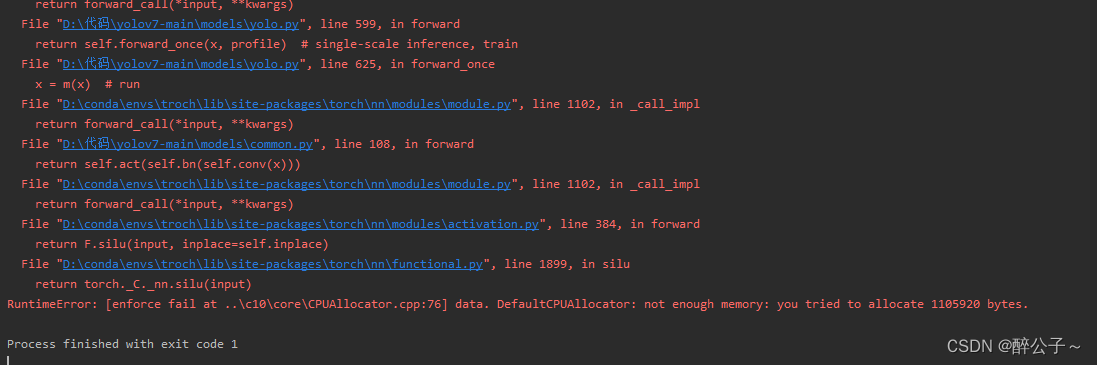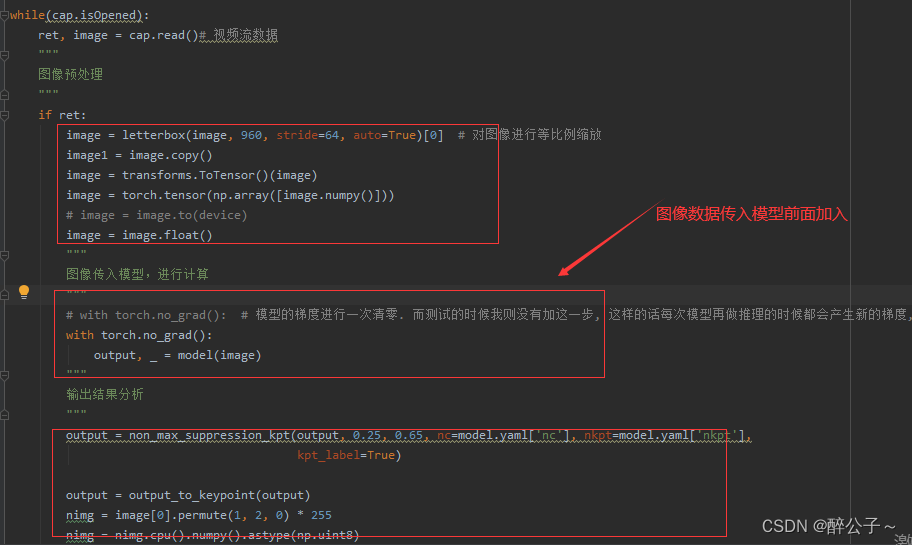# [Solved] RuntimeError: DefaultCPUAllocator: not enough memory: you tried to allocate 1105920 bytes.

Question

`RuntimeError: [enforce fail at ..\c10\core\CPUAllocator.cpp:76] data. DefaultCPUAllocator: not enough memory: you tried to allocate 1105920 bytes.`Today, when running yoov7 on my own computer, I used the CPU to run the test model because I didn’t have a GPU. I used the CPU to predict an independent image. There is no problem running an image. It is very nice!!! However, when I predict a video (multiple images), he told me that the memory allocation was insufficient,

`DefaultCPUAllocator: not enough memory: you tried to allocate 1105920 bytes.`,

Moreover, it does not appear after the second image is run. It appears when the 17th image is calculated. The memory can not be released several times later~~~~~~~~

analysis

In pytorch, a tensor has a requires_grad parameter, which, if set to True, is automatically derived when backpropagating the tensor. tensor’s requires_grad property defaults to False, and if a node (leaf variable: tensor created by itself) requires_grad is set to True, then all nodes that depend on it require_grad to be True (even if other dependent tensors have requires_grad = False). grad is set to True, then all the nodes that depend on it will have True (even if the other tensor’s requires_grad = False)

Note:

requires_grad is a property of the generic data structure Tensor in Pytorch, which is used to indicate whether the current quantity needs to retain the corresponding gradient information in the calculation. Taking linear regression as an example, it is easy to know that the weights w and deviations b are the objects to be trained, and in order to get the most suitable parameter values, we need to set a relevant loss function, based on the idea of gradient back propagation Perform training.

When requires_grad is set to False, the backpropagation is not automatically derivative, so it saves memory or video memory.

Then the solution to this problem follows, just let the model not record the gradient during the test, because it is not really used.

Solution:

Use `with torch.no_grad()`, let the model not save the gradient during the test:

``````with torch.no_grad():
output, _ = model(image) # Add before the image calculation
``````In this way, when the model calculates each image, the derivative will not be obtained and the gradient will not be saved!

Perfect solution!